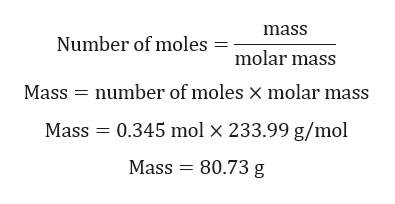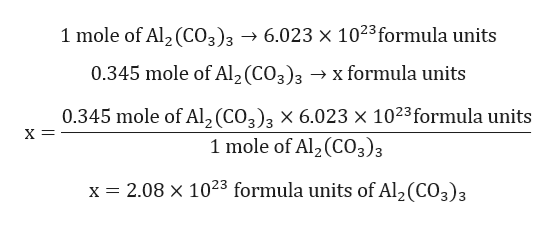# What is the mass of 0.345 mole of Al2 (CO3)? How many individual ionic formula units or particles does this amount represents?

Question
1 views

What is the mass of 0.345 mole of Al(CO3)? How many individual ionic formula units or particles does this amount represents?

check_circle

Step 1

Calculation mass of Al2(CO3)3:help_outlineImage Transcriptionclosemass Number of moles molar mass Mass = number of moles x molar mass Mass = 0.345 mol × 233.99 g/mol Mass = 80.73 g fullscreen
Step 2

Calculation for number of formula units:help_outlineImage Transcriptionclose1 mole of Al2 (CO3)3 → 6.023 × 1023 formula units 0.345 mole of Al, (CO3)3 → x formula units 0.345 mole of Al, (CO,), x 6.023 × 1023formula units 1 mole of Al2 (CO3)3 x = 2.08 × 1023 formula units of Al2 (CO3)3 fullscreen
Step 3

Calculation for number of Aluminium ions:

1 mole of Al2(CO3)3 contain 2 moles of Al.

0.345 mole of Al2(CO3)3 contain 0.69 moles of Al.

2.08×1023 formula units of Al2(CO3...

### Want to see the full answer?

See Solution

#### Want to see this answer and more?

Solutions are written by subject experts who are available 24/7. Questions are typically answered within 1 hour.*

See Solution
*Response times may vary by subject and question.
Tagged in

### Chemistry